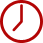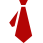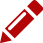<div style="margin:20px 0 0 200px"> To view the site, enable JavaScript by changing your browser options, then <a href="">Try Again</a>.</div>Duration

40 hoursEligibility

1. Good knowledge of Mathematics(Undergraduate)
2. Good understanding of Python programmingCourse Fees

Class Room Training: Rs.16,000/-
Inclusive of all taxes

Online: Rs.26,000/-
Inclusive of all taxes

Course Details

Introduction
• What is Machine Learning
• Why Machine Learning
• Application of Machine Learning
• Data and Data Preprocessing
Regression
• Simple Linear Regression
• Multiple Linear Regression
• Polynomial Regression
• Support Vector Regression (SVR)
• Decision Tree Regression
• Random Forest Regression
• Regression Models Performance
Classification
• Logistic Regression
• K-Nearest Neighbors (K-NN)
• Support Vector Machine (SVM)
• Kernel SVM
• Classification Models Performance
Clustering
• K-Means Clustering
• Hierarchical Clustering
Natural Language processing
Dimensionality Reduction
• Principal Component Analysis (PCA)
• Linear Discriminant Analysis (LDA)
• Kernel PCA
Model Selection and boosting
• Model Selection
• XGBoost
Project/AssignmentDuration

40 hoursEligibility

1. Basic Knowledge of Machine Learning
2. Good knowledge of Mathematics(Undergraduate)
3. Good understanding of Python programmingCourse Fees

Class Room Training: Rs.16,000/-
Inclusive of all taxes

Online: Rs.26,000/-
Inclusive of all taxes

Course Details

Introduction
• Introduction to Advanced Machine Learning
• Supervised Learning
• Unsupervised Learning
• Data and Data Preprocessing
Classification
• Naive Bayes
• Decision Tree Classification
• Random Forest Classification
• Classification Models Performance
Association Rule
• Aprion
• Eclat
Reinforcement Learning
• Upper Confidence Bound (UCB)
• Thompson Sampling
Deep Learning
• Artificial Neural Networks
• Convolutional Neural Networks
Probability
• Random Variable
• Mean and variance
• Probability
• Conditional Probability
• Bays Theorem
• Estimation
• Sampling
Machine Learning in Information Security
• Data Exploration
• Spam Filtering
Project/Assignment

Enroll Now

You're applying for Machine Learning Using Python
Rs. 16000 /- Inclusive of all taxes

Fields marked with * are mandatory.

Total Amount Payable: Rs. 16000/-